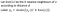# Understanding of K-Nearest Neighbours

K-NN is the most friendly algorithm in Machine Learning. K-NN can be used for both classification and regression problems. Predicting labels of data based on nearby neighbors, this concept is called Inductive Learning. We select the k- nearby neighbors to make a classification decisionMathematical Perspective for the definition of KNN

In the above equation, we can see that the predicted y label is the mode of its nearby neighbors. For example, a similar analogy is applied to K-NN, we have a set of numbers like {5,5,5,3,3,2} then the mode of the set is 5, so the new data point will be equal to 5.

To perform…## Sravani Subraveti

Hi!! I graduated Master’s from the University of Waterloo, an MEng in Electrical and Computer Engineering. Happiness is learning a new thing every day.